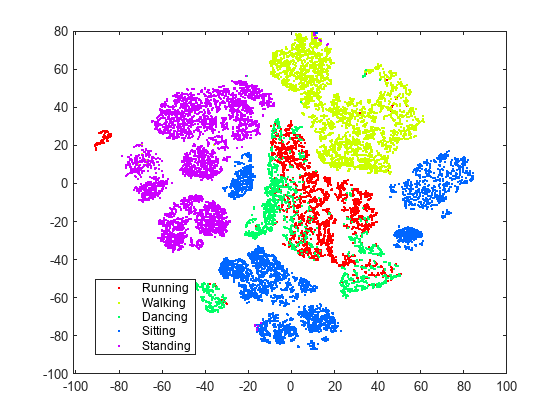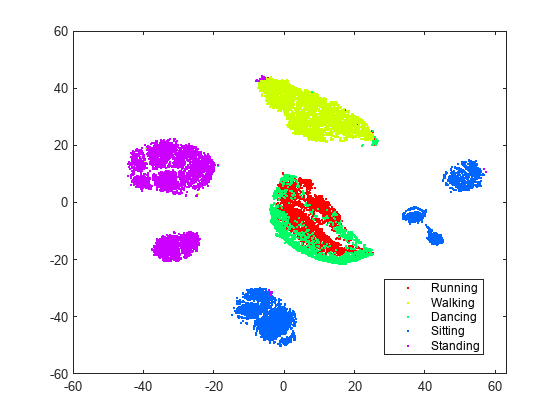# Visualize High-Dimensional Data Using t-SNE

This example shows how to visualize the MNIST data , which consists of images of handwritten digits, using the `tsne` function. The images are 28-by-28 pixels in grayscale. Each image has an associated label from 0 through 9, which is the digit that the image represents. `tsne` reduces the dimension of the data from 784 original dimensions to 50 using PCA, and then to two or three using the t-SNE Barnes-Hut algorithm.

### Obtain Data

Begin by obtaining image and label data from

http://yann.lecun.com/exdb/mnist/

Unzip the files. For this example, use the `t10k-images` data.

```imageFileName = 't10k-images.idx3-ubyte'; labelFileName = 't10k-labels.idx1-ubyte';```

Process the files to load them in the workspace. The code for this processing function appears at the end of this example.

`[X,L] = processMNISTdata(imageFileName,labelFileName);`
```Read MNIST image data... Number of images in the dataset: 10000 ... Each image is of 28 by 28 pixels... The image data is read to a matrix of dimensions: 10000 by 784... End of reading image data. Read MNIST label data... Number of labels in the dataset: 10000 ... The label data is read to a matrix of dimensions: 10000 by 1... End of reading label data. ```

### Reduce Dimension of Data to Two

Obtain two-dimensional analogues of the data clusters using t-SNE. Use PCA to reduce the initial dimensionality to 50. Use the Barnes-Hut variant of the t-SNE algorithm to save time on this relatively large data set.

```rng default % for reproducibility Y = tsne(X,'Algorithm','barneshut','NumPCAComponents',50);```

Display the result, colored with the correct labels.

```figure numGroups = length(unique(L)); clr = hsv(numGroups); gscatter(Y(:,1),Y(:,2),L,clr)```t-SNE creates clusters of points based solely on their relative similarities that correspond closely to the true labels.

### Reduce Dimension of Data to Three

t-SNE can also reduce the data to three dimensions. Set the `tsne` `'NumDimensions'` name-value pair to `3`.

```rng default % for fair comparison Y3 = tsne(X,'Algorithm','barneshut','NumPCAComponents',50,'NumDimensions',3); figure scatter3(Y3(:,1),Y3(:,2),Y3(:,3),15,clr(L+1,:),'filled'); view(-93,14)```Here is the code of the function that reads the data into the workspace.

```function [X,L] = processMNISTdata(imageFileName,labelFileName) [fileID,errmsg] = fopen(imageFileName,'r','b'); if fileID < 0 error(errmsg); end %% % First read the magic number. This number is 2051 for image data, and % 2049 for label data magicNum = fread(fileID,1,'int32',0,'b'); if magicNum == 2051 fprintf('\nRead MNIST image data...\n') end %% % Then read the number of images, number of rows, and number of columns numImages = fread(fileID,1,'int32',0,'b'); fprintf('Number of images in the dataset: %6d ...\n',numImages); numRows = fread(fileID,1,'int32',0,'b'); numCols = fread(fileID,1,'int32',0,'b'); fprintf('Each image is of %2d by %2d pixels...\n',numRows,numCols); %% % Read the image data X = fread(fileID,inf,'unsigned char'); %% % Reshape the data to array X X = reshape(X,numCols,numRows,numImages); X = permute(X,[2 1 3]); %% % Then flatten each image data into a 1 by (numRows*numCols) vector, and % store all the image data into a numImages by (numRows*numCols) array. X = reshape(X,numRows*numCols,numImages)'; fprintf(['The image data is read to a matrix of dimensions: %6d by %4d...\n',... 'End of reading image data.\n'],size(X,1),size(X,2)); %% % Close the file fclose(fileID); %% % Similarly, read the label data. [fileID,errmsg] = fopen(labelFileName,'r','b'); if fileID < 0 error(errmsg); end magicNum = fread(fileID,1,'int32',0,'b'); if magicNum == 2049 fprintf('\nRead MNIST label data...\n') end numItems = fread(fileID,1,'int32',0,'b'); fprintf('Number of labels in the dataset: %6d ...\n',numItems); L = fread(fileID,inf,'unsigned char'); fprintf(['The label data is read to a matrix of dimensions: %6d by %2d...\n',... 'End of reading label data.\n'],size(L,1),size(L,2)); fclose(fileID); ```

### References

 Yann LeCun (Courant Institute, NYU) and Corinna Cortes (Google Labs, New York) hold the copyright of MNIST dataset, which is a derivative work from original NIST datasets. MNIST dataset is made available under the terms of the Creative Commons Attribution-Share Alike 3.0 license, https://creativecommons.org/licenses/by-sa/3.0/